MEMO 2008 ekipno problem 7

Kvaliteta:
Avg: 2,5
Težina:
Avg: 5,5
Let$ABC$ be an acute-angled triangle. Let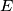$E$ be a point such$E$ and$B$ are on distinct sides of the line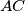$AC$, and$D$ is an interior point of segment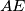$AE$. We have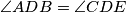$\angle ADB = \angle CDE$,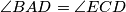$\angle BAD = \angle ECD$, and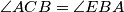$\angle ACB = \angle EBA$. Prove that$B$,$C$ and$E$ lie on the same line.
Izvor: Srednjoeuropska matematička olimpijada 2008, ekipno natjecanje, problem 7